# Möbius Assessment Question Types#### Möbius Assessment has many question types for both technical and non-technical subjects, including:

For information on other question types, including true/false, matching, chemical equation questions, and more, refer to the Möbius Assessment Instructor Help.

### Mathematical Free Response

Möbius Assessment allows you to ask free-response questions, where students provide their mathematical solution the same way they would on paper.
• Natural math notation is used in both the question text and student response.
• Easy-to-use equation editor makes it simple to enter mathematical expressions for both students and instructors.
• Questions are graded for mathematical equivalence. Where appropriate, responses do not need to be identical to the solution to be graded as correct; they just need to be equivalent.  For example, if the correct answer is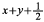, Möbius Assessment will also accept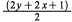, and if the answer is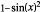, Möbius Assessment will also accept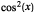. Alternatively, you can require responses to be in a particular form.
• Open-ended questions can have infinitely many answers. With Möbius Assessment you can ask questions for which you cannot know in advance how the student will respond, and Möbius Assessment can still grade their responses automatically.  You can ask questions such as “Give an example of a function that has a maximum at x = 0,” “List two composite numbers that have no factors in common,” or “Give an example of an invertible matrix.”
• Extensive mathematical knowledge coming from Maple™ means Möbius Assessment can automatically grade questions from virtually any area of mathematics.

Adaptive questions provide students another chance when they give an incorrect response. Knowing the student is having trouble, the question can be adapted to:
• Let the student try a simpler version of the question before retrying the original problem.
• Walk the student through the problem one step at a time.
• Try the same question again for reduced credit.
• Present whatever other approach the instructor feels is appropriate.

With adaptive questions, instructors can control the behavior of the question, including:

• When a new version or solution step appears.
• The number of attempts that are allowed at each stage.
• How much credit the student gets, including partial credit for incomplete answers and penalty deductions for repeated attempts.
• If the correct answer should appear after the problem has been attempted the maximum number of times.
• When to move onto the next question in the assignment.

### Graph Sketching

With the Sketch question type, instructors can ask students to sketch graphs, and Möbius Assessment grades those graphs automatically.
• Students answer a question by sketching a plot.
• Parabolas, exponentials, asymptotes, lines, points, piecewise, open and closed intervals, and regions are all supported.
• Students create plots by simply clicking on key points of the plot in the graph area, such as specifying two points to draw a line, or the vertex and one other point to create a parabola.
• Plot control points can be moved interactively by dragging, which automatically adjusts the rest of the curve.
• Graph sketching questions can include curves that are part of the question itself, such as showing a line and asking the student to draw a tangent to that line through a given point.
• Hundreds of customizable graph sketching questions are available in the Möbius Cloud.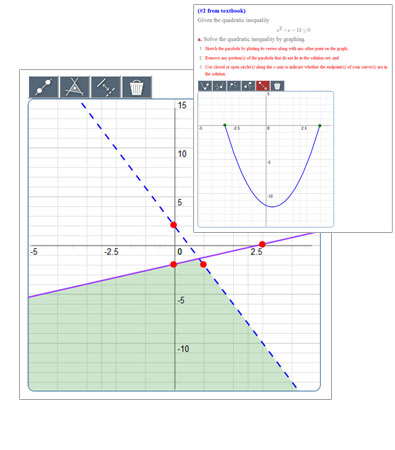### Free Body Diagrams

Instructors in engineering and science can ask questions that require students to draw free body diagrams, and Möbius Assessment automatically grades the results.
• Students answer a question by drawing force arrows at appropriate locations and angles on an image illustrating the problem, such as a ramp, pulley, cart, truss, bridge, or other statics or dynamics problems.
• The student must specify the type of each force, such as gravity, normal, or friction, and show the direction of each force in relation to the object.
• The direction of each force is assessed within a specified tolerance.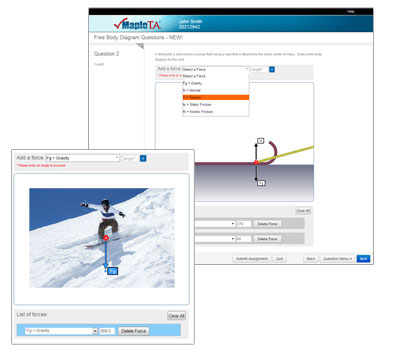Instructors can include interactive Maple™ documents (“Math Apps”) in Möbius Assessment questions, and Möbius Assessment will grade the interactions with student has with the Math App.
• With gradeable Math Apps, instructors can provide students with specialized calculators, ask them to manipulate parameters until they have found the desired solution, give them interactive plots to explore, and much more.
• Students interact with the Math App directly inside Möbius Assessment.
• Möbius Assessment can automatically grade interactive Math Apps by examining what the student did with the application, such as moving a slider that controls a plot to a particular value.
• If there is more than one correct answer, Möbius Assessment can analyze the response mathematically to assign an appropriate grade.
• Grading code can be placed either in the Möbius Assessment question definition or within the Maple™ document itself
• Math App questions can contain algorithmically-generated variables so each student receives a different version of the Math App question### Numeric response with margin-of-error

• The response must match the correct answer to within a specified tolerance
• Acceptable tolerance can be set by the instructor
• Converts automatically between  scientific notation and decimal notation, so both forms are accepted when desired
• Supports the use of units, performing necessary conversions while evaluating the response

### Multiple Choice

• Algorithmically generated variables can be used in both question and answers
• You can automatically permute the possible answers so different students see the answers in different orders
• Questions and answers can include images, videos, audio clips, and more,  including algorithmically generated plots that change with each question
• Supports both multiple choice and multiple selection, where more than one answer must be chosen to answer the question

### Fill in the blank

• Blanks can represent a single word or a phrase
• Blanks can be filled by entering in the missing word or phrase, or by choosing them from a list
• Multiple correct answers can be provided to account for alternate spellings and phrasings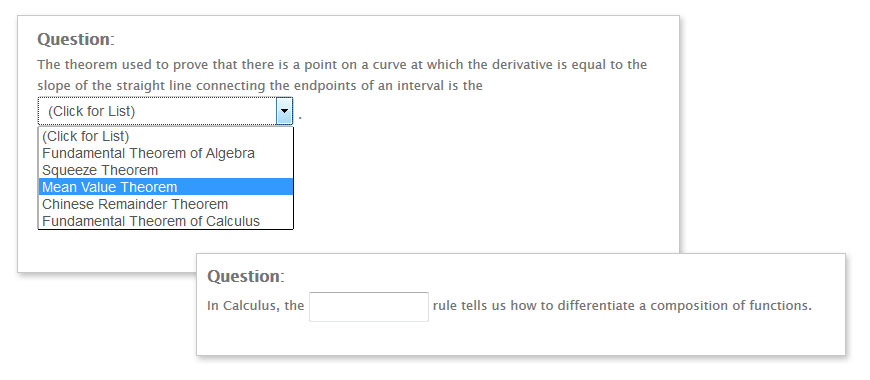### Essay

• Used for long answer responses
• Can be used for mathematical proofs, long answer questions, essays, and more
• Responses are sent to the instructor automatically for manual grading
• Instructors can provide detailed feedback by placing comments at selected points inside the essay
• Instructors choose from over  40 common phrases, such as “spelling” and “sentence fragment”, or can add their comments
• Essay questions are tagged in the gradebook for easy identification and grade entry

### Clickable Image

• Ask students to identify an element of an image by clicking on it. For example, a clickable image question could be used to ask students to identify a specific element of a cell diagram, the focus of an ellipse, or the center of gravity of a gymnast.
• The image includes multiple hot spots for the student to choose from, chosen by the instructor.
• Any image can be used in the question.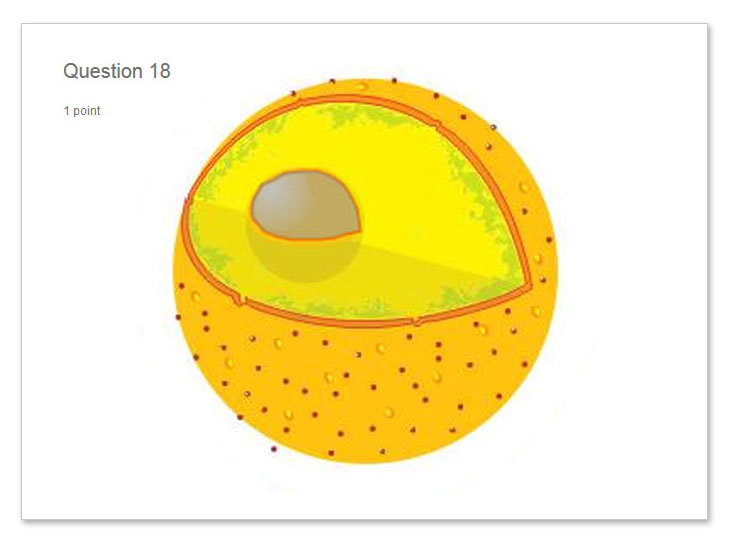### Are you a student?

Retrieve your student access below or browse our
support documents if you have any questions.

Not a student?

Continue to DigitalEd.com